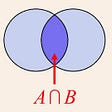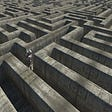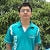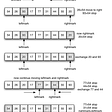# Introduction:

Dinic’s algorithm is an algorithm made to solve a type of problem called the max-flow problem. To talk about the max-flow problem, let us assume a graph with nodes and weighted edges. The weights in the edges represent the flow the edge can take, or one can also say that it represents how much stuff that line can carry.

Like for example, there are only some types of roads in some countries that do not support the movement of heavy vehicles. Unmetalled roads do not support the movement of cars and bikes, and a lot more. So what I want to know after I solve the max flow problem is that, what are the best types of vehicles I can use, so that I can go from my source to my destination on that same vehicle throughout my journey.

# Let’s know some things:

The first thing to know is, of course, that it is a single-source single-sink problem. Another thing to know about is “Residual Capacity”. The Residual Capacity is simply the weight of the edge at a particular moment of time. Another thing to mention is something called “Augmenting Level Path”. This is simply the path made from the source to the destination from the tree-version of the graph made intuitively by the Breadth-First Search approach.

Let us summarise the same with the picture given below:

# The Explanation:

Let us take the example of the graph we took in the summary. We also find that the augmenting level path for the graph is 1–2–5–7. So for this case, min_cap = 2. So what shall we do is that we will deduct 2 from each edge in the path, and add a reverse edge of weight 2, in the graph, like this:

Also, let us add 2 to the max_flow.

max_flow = 0 + 2 = 2

So with the new graph formed we need to make a new tree for the augmenting level path, which is like this:

So the new augmenting level path we found is 1–3–6–7. min_cap = 2 for this case also. So let us do the same we did before:

Again, let us add 2 to the max_flow.

max_flow = 2 + 2 = 4

Again let us make a new tree for the augmenting level path, like this:

So the new augmenting path is 1–2–4–6–7. But for this case, min_cap is 1. So let us remove 1 from the path and add reverse edges appropriately, like this:

Let us add 1 to the max_flow.

max_flow = 4 + 1 = 5.

On examining further, we do not find any other augmenting level path, in this graph that goes from the source to the destination. So we conclude that we have found the max_flow for this particular graph.

# References:

Thank you !!!

--

--

--

Love podcasts or audiobooks? Learn on the go with our new app.

## Five Steps to Better Data Quality## Is it going to rain today?## Vector similarity search with Qdrant## Trawling Twitter for Trollish Tweets## Estimating the long-run value we give to our users through experiment meta-analysis## Monitoring Home Air Quality With a Raspberry Pi## Scalable Deep Learning on Parallel and Distributed Infrastructures## Multistage Graph Problem## Employing Field Normalization To Resolve Data Quality Issues In ServiceNow## LIGHTING THE FUTURE WITH FLARE## Quick Sort — [Introduction to Algorithms]